Truck inspections 2000 [German version]

 On 13th July 2000, a truck inspection operation was carried out at the Plochinger interchange near Esslingen. Officials from Esslingen traffic police and load securing experts from transport insurers within GDV inspected 23 vehicles in six hours. Serious load securing deficiencies were identified on 19 trucks, with slight deficiencies being found on 4 vehicles.

 A brief selection of pictures and explanations relating to the load securing inspection operation: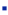Double I beamsConcrete and metal componentsDumpster container (1)Dumpster container (2)PackagesSmall excavatorTransport of double I beams:

Deficiencies: Large gaps in the load, no load securingFigure 1Figure 2Figure 3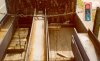Figure 4Figure 5

The only economic way of securing steel beams in the direction of travel is by providing a tight fit with headboards which have a sufficient loading capacity. If the headboard does not have sufficient loading capacity or if gaps in the load are necessary for load distribution reasons, one possible option is to use headboards which can be inserted in stanchion pockets, as shown on page 204 of the GDV Cargo Securing Manual. Such headboards with sufficient loading capacity may be inserted as required in the stanchion pockets or, if constructed with sleeves, slipped over the stanchions. They provide a secure, tight fit which ensures that the load needs no further securing measures in the longitudinal direction.

This extremely inadequate load securing can be improved by subsequently filling in the gap between the load and headboard or by directly securing the end face of the double I beams with an overhead loop.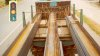Figure 6Figure 7

Figure 6 clearly shows the path of the belt around the central stack of the load. On page 293 of the GDV Cargo Securing Manual , loop lashings have been drawn in to clarify the securing of a comparable load. Provided that the belt material used cannot be damaged by the sharp corners or that suitable belt protectors are used, each belt may now be loaded up to its lashing capacity (LC on the blue label on the belt).

Supposing that a belt material has been used which can be loaded to 2,000 daN in a straight pull and that the two load securing points on the vehicle are of equal or greater strength, the strength or lashing capacity (LC) is calculated as follows:

The belt is being used as a loop lashing to secure the load to the side. If the belt is fixed to two different load securing points, it can withstand a securing force of twice the belt’s LC. The LC is only reduced by the angle α (approx. 45°) of the upper section of the belt (passing over the load stack, see diagram 1). The lower section of the belt has virtually 100% of its securing force.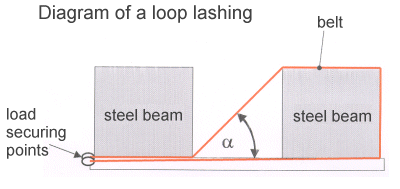Diagram 1

The following diagram 2 and a worked example are intended to provide a simplified illustration of the amount by which the securing force is reduced as a function of the angle α.

In order to simplify the calculation conceptually, it is assumed that the angle α (45°) applies to both sections of the belt: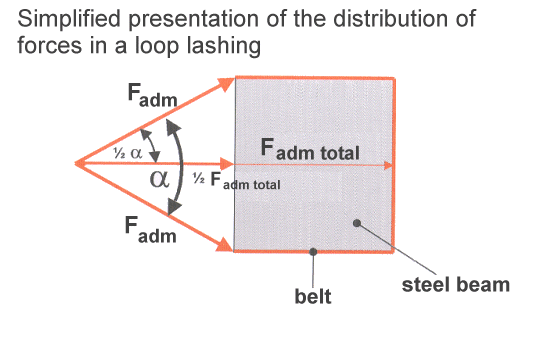Diagram 2

Angle α = 45°

Admissible lashing force = Fadm = 2000 daN

The following function is obtained: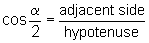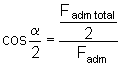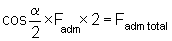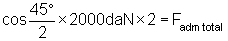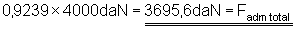The result states that, due to the angle (cos 45°/2) only 92.39% of the actual securing force remains in effect.

In loop lashings, one section of the belt is usually applied at an angle of 40 to 60°. This means that the 100% securing force of a belt is reduced as a function of the angle to, for example, (cos 40°/2) 94% or (cos 60°/2) 87% and, in extreme cases (cos 90°/2) 71%.

Since two sets of loop lashings (one to the left and one to the right) must always be applied to the front and rear load stacks, four individual loop lashings are required to secure the central load stack.

The following worked calculation shows that at a friction coefficient (metal-wood) of µ = 0.3 and a maximum sideways acceleration of 0.5 g (g = acceleration due to gravity = 9.81 m/s²), a 37 metric ton load stack can be secured against sideways inertia forces with two loop lashings on each side:

Root: LW = load weight/weight-force (1 metric ton or 1000 kg correspond to 1000 daN)
 Acceleration force: Sideways inertia force (0.5 g) = 50% of load weight = 0.5 x LW daN
 Securing force: Metal-wood friction force (µ = 0.3) = 30% of load weight = 0.3 x LW daN Plus two loop lashings in accordance with the above worked example, each with an admissible lashing force of 3695.6 daN = 7391.2 daN
 Equation: Acceleration force = securing force Sideways inertia force = friction force + 2 loop lashings 0.5 x LW daN = 0.3 x LW daN + 7391.2 daN Solve for LW (0.5 – 0.3) x LW daN = 7391.2 daN LW daN = 7391.2 daN / 0.2 LW daN = 36956 daN ≈ 36956 kg ≈ 37 metric tons

Another worked example is intended to demonstrate that far lower securing forces are achieved by tie-down lashing:

Since, when applying tie-down lashing, prestressing must not exceed half the strength of the lashing belt, the admissible lashing force (LC) of 2000 daN per belt end falls to a prestressing force of just 1000 daN per belt end. However, unless long-lever ratchet tensioners are used, a maximum prestressing force of only 400 daN per belt end can be assumed. Taking account of a lashing angle of 45° on each side (which can be disregarded when calculating sideways inertia force), a friction coefficient (metal-wood) of µ = 0.3 and sideways acceleration of 0.5 g, only 4.8 metric tons can be secured with 4 tie-down lashings. Moreover, tie-down lashings require constant relashing.

Root: LW = load weight/weight-force (1 metric ton or 1000 kg correspond to 1000 daN)
 Acceleration force: Sideways inertia force (0.5 g) = 50% of load weight = 0.5 x LW daN
 Securing force: Metal-wood friction force (µ = 0.3) = 30% of load weight = 0.3 x LW daN plus 4 overlashings each with a prestressing force of 400 daN per 2 belt ends and a friction coefficient of µ = 0.3 (4 x 400 x 2 x 0.3) = 960 daN
 Equation: Acceleration force = securing force Sideways inertia force = friction force + 4 overlashings (tie-down lashings) 0.5 x LW daN = 0.3 x LW daN + 960 daN Solve for LW (0.5 – 0.3) x LW daN = 960 daN LW daN = 960 daN / 0.2 LW daN = 4800 daN ≈ 4800 kg ≈ 4.8 metric tons

Back to beginningConcrete and metal components on pallets:

Deficiencies: Large gaps in the load, no load securingFigure 8Figure 9

Back to beginningTransport of a dumpster container loaded with scrap metal (1):

Deficiencies: Wrong means of transport, excessive load, deficient load securing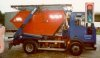Figure 10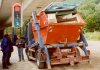Figure 11Figure 12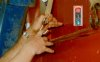Figure 13

Due to their shape and construction, dumpster containers are of course capable of securing bulk materials such as scrap lumber, sand, stones etc.. They are not designed for carrying general load and thus do not have load securing points for attaching belts, chains or the like.

It proved impossible to make the load secure as it was, so the overflowing container had to be removed from the vehicle and a second one fetched which could accommodate the load.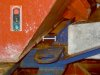Figure 14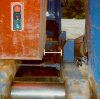Figure 15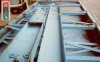Figure 16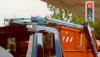Figure 17

Overall assessment:

Even the gentlest braking in traffic would have set the pieces of steel scrap in motion over the top of the vehicle, so causing considerable damage.

Back to beginningTransport of a dumpster container loaded with scrap lumber (2):

Deficiency: Inadequate or absent load securing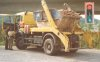Figure 18Figure 19Figure 20

Back to beginningTransport of packages:

Deficiency: Inadequate load securing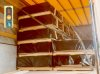Figure 21Figure 22

Figures 21 and 22 show the (additional) subsequently applied securing. Load securing was improved with loop lashings (belt 1 and belt 2) over the end faces of the load block, while another belt (belt 3) was used for tie-down lashing.

Prior to this, the load was secured as follows: packages, each weighing 800 kg, were loaded onto a semitrailer with a tarpaulin body. The vehicle had been partially unloaded, which meant that loading of the vehicle was one-sided. Moreover, the load was not secured to the front, except for two tie-down lashings which had been tightened over the two load stacks. Only the bottommost two packages were in each case secured sideways by a tight fit against the side walls.

The following rough calculation shows that two tie-down lashings did not provide adequate forwards securing:

The following pairs of materials, wood-wood or wood-metal on the loading area and wood-plastic film in the stacked packages, must be taken into consideration when determining frictional securing. Friction is estimated to amount to at most 30% of the load weight. The maximum acceleration force applying in the direction of travel (horizontal inertia force) is assumed to be 80% of load weight, while the maximum sideways acceleration forces is assumed to be 50% of load weight (corresponding to 0.8 and 0.5 g respectively). Prestressing is assumed to be 400 daN on each side of the two overlashings, although the actual measured prestressing force was only 100 daN per side (400 daN could be achieved by retightening). The diminished action of the tie-down lashing due to the angle on the right hand side is disregarded (at 45Â° approx. 30% in the longitudinal direction).

Forwards securing: With a load weight of (5 x 800 kg = 4000 kg) 4000 daN and taking the maximum horizontal inertia force into account (0.8 x 4000 daN), 3200 daN of securing force must be applied. Friction force (0.3 x 4000 daN) first of all provides 1200 daN. A further 480 daN are provided by two overlashings with prestressing of 400 daN on each side (2 stressed sides) and a friction coefficient of 0.3 (2 x 400 daN x 2 x 0.3 = 480 daN). In other words, there was a 1520 daN shortfall in securing force for the five packages.

Sideways securing: since the bottom two packages of each stack were secured to the side by the side walls, only the securing for the upper three packages need be determined. With a load weight of (3 x 800 kg = 2400 kg) 2400 daN and taking the maximum sideways inertia force into account (0.5 x 2400 daN), 1200 daN of securing force must be applied. Friction force (0.3 x 2400 daN) first of all provides 720 daN. A further 480 daN are provided by two overlashings with prestressing of 400 daN on each side (2 stressed sides) and a friction coefficient of 0.3 (2 x 400 daN x 2 x 0.3 = 480 daN). In other words, the securing forces are just sufficient for the three packages.

The load could have been correctly secured as follows: a forklift truck ought to have been used to transfer the top package from the left hand stack onto the right hand stack and to place another package from the left hand package centrally across the two stacks. Sideways securing could have been provided by tie-down lashing or loop lashings and forwards securing by two direct lashings passed crosswise over the end face of the load.

Back to beginningTransport of a small excavator:

Deficiency: Inadequate load securing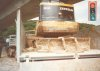Figure 23Figure 24Figure 25Figure 26

By changing over from tie-down lashing to direct lashing, which entails no additional labor, the securing action could be raised from virtually zero to almost 100% of that required:Figure 27Figure 28

Back to beginning